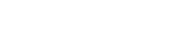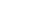# Mathematics Questions and Answers - Form 1 End Term 1 Exams 2023

QUESTIONS

1. Write in words 27707807. (1mk)
2. The sum of three consecutive whole numbers is 84. Find the numbers. (3mks)
3. A number n is such that when it is divided by 27, 30 or 45, the remainder is 3. Find the smallest value of n. (3mks)
4. Use the number line to perform the following operations.
1. (-10) − (−3) (1mk)
2. (+1)−(−8) (1mk)
3. (−3)+(−4) (1mk)
5. If x=−2, y=−6 and z=4, find the values of:
1. 2y−3x+z (2mk)
2. 4xy  (2mks)
z
6. Solve(3mks)
7. A farmer has 165 orange trees on his farm. 38 trees have 97 oranges each, 79 trees have 176 oranges each, the rest have 59 oranges each. How many oranges are there altogether? (3mks)
8. Express as a single fraction (3mks)
9. Given that= 1a/b. Find the value of a and b (3mks)
10. On a certain day, a student measured the temperature inside a deep freezer and found that it was −3°C while the room temperature was 24°C. What was the temperature difference between the room and the deep freezer? (2mks)
11. Using tables find the:
1. Square of 4.973 (2mks)
2. Square root of 0.146 (2mks)
12. An integer p is two-thirds of another and their difference is 10. Find the two integers.(3mks)
13. Check whether 7544 is divisible by 6 (3mks)
14. Write the following in standard form.
1. 0.00121
2. 4521.021
15. Evaluate  0.17 × 1.05 + 0.32  correct to three decimal places. (4mks)
4.5 × 0.08 − 0.089
16. Find the square root of 1764 using factor method. (3mks)
17. Remove the brackets and simplify.(3mks)
2b + a[3 − 2(a − 5)]## MARKING SCHEME

1. Twenty seven million, seven hundred and seven thousand, eight hundred and seven. √
2. X,  x+1,  x+2 √
X+x+1+x+2=84
3x+3=84
3x=84−3
3x=81
X=27√
X+1=28
X+2=29√
3. L.C.M of 27, 30 and 45=270√
n = 270+3√
n = 273√
4.
1. =−7
2. =+9
3. = −7
5.
1. = 2(−6) – 3(−2) + 4 = −2 substitution 1mk
2. 4(–2)(–6)48 = 12
4             4
6. = 2/5 of {32/5 − 1 × 1/5}√
= 2/5 of {31/5}√
= 62/25
=2 12/25
7. 38,  97 oranges each
79,   176 oranges each
Rest  165 − (38 + 79) = 48
Total oranges =(38×97) + (79×176) + (48×59)
=20422 oranges
8. (x+1) − (x−1) =  3(x+1) −2(x−1)
2           3                6
= 3x + 3−2x + 2
6
= x + 5
6
9.     r = 1.050505√
10r = 10.50505
100r = 105.0505
100r − r = 105.0505 − 1.0505
99r  = 104
r = 104/99  √
r = 15/99
a = 5,   b = 99√
10. 24 − (−3) = 24 + 3√
= 27℃√
11.
1. 24.701 add 30 = 24.731
2. 0.146 = 14.6× 10−2
square root  = 3.821× 10-1
=0.3821
12. a, p = 2/3a√
a − 2/3a = 10√
3a − 2a=30
a = 30,   p = 20√
13. 7544 is divisible by 2 since the last digit is an even number.
7+5+4+4 = 20
7544 is not divisible by 3 since the sum of its digits is not divisible by 3
7544 is not divisible by 6
14.
1. 0.00121 =1.21× 10−3
2. 4521.021 = 4.521021 × 103
15. 0.17 × 1.05 + 0.32
4.5 × 0.08 − 0.089
Numerator = 0.17×1.05+0.32
= 0.1785+0.32
= 0.4985 √
Denominator = 4.5 × 0.08 − 0.089
= 0.36 − 0.089
= 0.271√          BODMAS  M1
0.4985 = 1.839√
0.271
16. 1764 = 2×882
= 2×2×441      M1
= 2×2×3×147\
= 2×2×3×3×49
= 2×2×3×3×7×7
=22 × 32 × 72          M1
√1764 = 2×3×7 = 42            A1
17. 2b + a [3 − 2(a − 5)]
=2b + a(a − 2a+10)√
=2b + a(−a+10)√
=2b − a2 + 10a
= −a2 +10a + 2b√

• ✔ To read offline at any time.
• ✔ To Print at your convenience
• ✔ Share Easily with Friends / Students